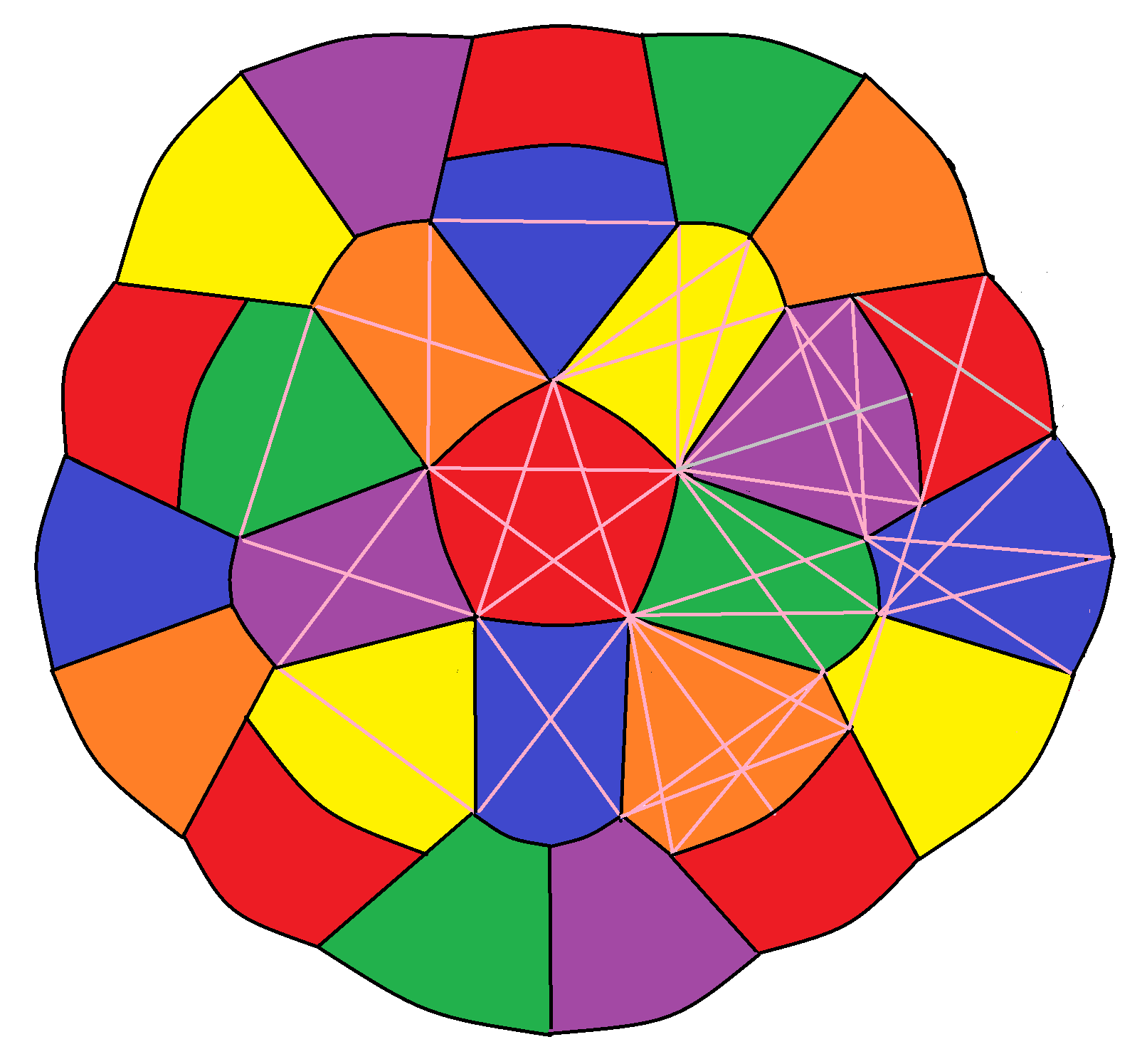# 6-colourings of subsets of the plane

There has been further recent activity on the Chromatic Number of the Plane problem, with an eleventh research thread being spawned. Philip Gibbs has been able to 6-colour a large disc (with diameter slightly greater than 4), and Aubrey de Gray has remarked that it can be enlarged slightly further still:An infinite strip of width$\sqrt{3} + \frac{1}{2} \sqrt{15}$ can similarly be 6-coloured in a relatively simple way.

### What about the whole plane?

Interestingly, it has been shown that any tile-based 6-colouring of the plane is critical in the sense that the maximum diameter of any tile must be equal to the minimum separation between similarly-coloured tiles; there is no room for manoeuvre. Moreover, this means that it is insufficient to simply specify the colours of the tiles themselves; it is necessary to also colour the (measure-0) vertices and edges where they meet!

This entry was posted in Uncategorized. Bookmark the permalink.

### 2 Responses to 6-colourings of subsets of the plane

1.David Speyer says:

“it is insufficient to simply specify the colors of the tiles themselves; it is necessary to also color the (measure-0) vertices and edges where they meet!” That’s wrong, actually. Someone (maybe Terry Tao) had a very clever argument early on in the discussions that, if you can k-color the plane except for a set of measure 0, then you can k-color the plane.

I can’t find the argument to link to right now, but I remember it: Let U be a subset of R^2 whose complement has measure 0, such that the unit distance graph of U can be k-colored. Let G be any finite unit distance graph. Then a random translate of G will, with probability 1, lie in U. So G can be k-colored. But the De Bruijn–Erdos theorem states that, if every finite subgraph of a graph can be k-colored, then the whole graph can be k-colored. So this would show that the unit distance graph of R^2 can be k-colored.

•apgoucher says:

That’s a beautiful proof! Observe that the resulting k-colouring of the plane is no longer guaranteed to be tile-based, though, so it’s entirely possible that the following are simultaneously true:

(a) There exists a tile-based 6-colouring of an open subset of R^2 with measure-0 complement;
(b) There does not exist a tile-based 6-colouring of R^2;
(c) There does exist a nonconstructive 6-colouring of R^2 (assuming choice).

The proof you mentioned entails (a) –> (c), but not that (a) –> ¬(b).